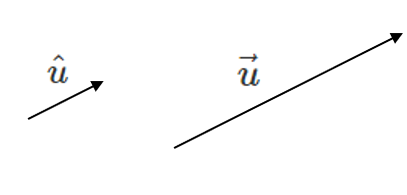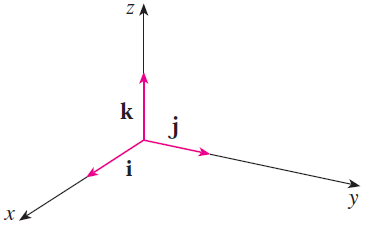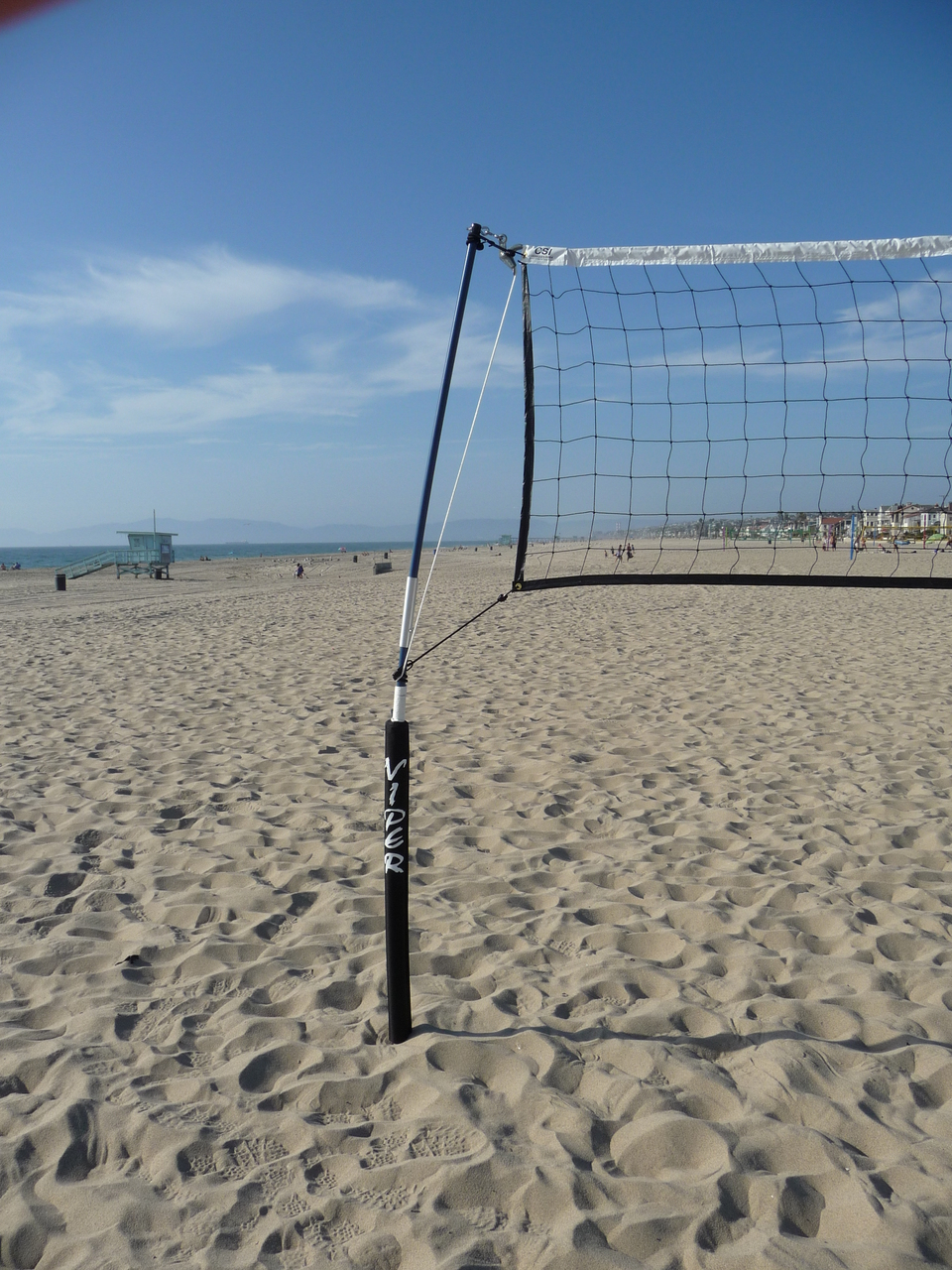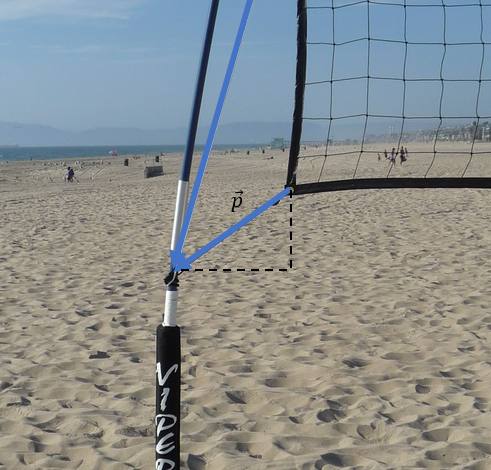# Unit Vectors

A Unit Vector has a magnitude of 1 and is denoted like $$\hat{u}$$. You can make a unit vector in the same direction of a vector by dividing by the magnitude:

$$\hat{u} = \frac{\vec{u}}{|\vec{u}|}$$

By multiplying a vector by a scalar equal to the magnitude, we are able to stretch the vector until it has a magnitude of 1 but keep the direction. You can say that the vecetors are Collinear or Parallel Vectors .Write a unit vector in the direction of $$\vec{u}= 10 \; \; \; 70^\circ \; S \; of \; W$$.

Write a unit vector in the direction of $$\vec{u} = \left<4, -2\right>$$.

The Standard Basis unit vectors are in the direction of the the x, y and z axes:

$$\hat{\imath} = \left<1,0,0\right>$$

$$\hat{\jmath} = \left<0,1,0\right>$$

$$\hat{k} = \left<0,0,1\right>$$Later, we will see how we can use these unit vectors to create linear combinations of other vectors.

##### Try these questions:

We can also use Unit Vectors to convert a Geometric Vector to an Algebraic Vector when there is information about how the vector interacts. For example, consider the force along a rope holding up a beach volleyball net. The force acts in the direction of the rope. If we know the magnitude of the force, we can convert it into an Algebraic Vector.First, we measure the x and y-components of the rope. We can make a position vector $$\vec{p}$$ which represents the a vector along the physical rope:

$$\vec{p} = \left<-10, -10 \right> [cm]$$

Next, let's find the Unit Vector $$\hat{p}$$:

$$\hat{p} = \frac{\vec{p}}{|\vec{p}|} = \frac{1}{\sqrt{(-10)^2+(-10)^2}} \vec{p}$$

$$\hat{p} = \frac{1}{10\sqrt{2}} \left<-10, -10 \right>$$

Now, the force in the rope will also run in the direction of the rope. That means:

$$\hat{F} = \hat{p} = \frac{1}{10\sqrt{2}} \left<-10, -10 \right>$$

We know that the force vector is:

$$\vec{F} = \hat{F} |\vec{F}|$$

That is to say, the force vector has a magnitude $$|\vec{F}|$$ and runs in the direction of $$\hat{F}$$.

If we know the magnitude is $$50 [N]$$ then the vector is:

$$\vec{F} = \hat{F} |\vec{F}|$$

$$\vec{F} = \frac{1}{10\sqrt{2}} \left<-10, -10 \right> (50)$$

$$\vec{F} = \left<\frac{-500}{10\sqrt{2}}, \frac{-500}{10\sqrt{2}} \right>$$

$$\vec{F} = \left<\frac{-50}{\sqrt{2}}, \frac{-50}{\sqrt{2}} \right> \; [N]$$

Remeber, the $$\frac{1}{10\sqrt{2}}$$ and $$50$$ are scalar multiples.

We can double check the magnitude of $$F$$:

$$|\vec{F}| = \sqrt{\left(\frac{-50}{\sqrt{2}}\right)^2 + \left(\frac{-50}{\sqrt{2}}\right)^2}$$

$$|\vec{F}| = \sqrt{\frac{50^2}{2} + \frac{50^2}{2}}$$

$$|\vec{F}| = \sqrt{50^2}$$

$$|\vec{F}| = 50 \; [N]$$